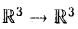【站僕】摩檸Morning>試卷(2016/09/10)

# 94 年 - 94 淡江大學 轉學考 線性代數#56042

【非選題】
1.1. (5 points) Let A and B be n x n matrices. IsIf so, prove it; if not, give a counterexample(反例）and state ujicler what conditions the equation is true.

【非選題】
2.2. (5 points) Let a, b and c be real numbeis(實數)such that abc ≠ 0. Prove that the plane ax + by -f- cz = 0 is a subspace(子空間)of【非選題】
3.3. (10 points) Let T :be a linear transformatioa. If T([l,0,0]) = [-3,1], T([0，l,0]) = [4,-1], and T([0,-i, 1]) = [3,5], find T[-1,4,2]).

【非選題】
4.
4. Let L :be a linear transibnnation defined by L【題組】 (a) (5 points) Find the standard matrix representation(標準矩陣表示式)of L.

【非選題】
5.【題組】(b) (5 points) Sliow that L is invertible(可逆）.

【非選題】
6.【題組】(c) (5 points) Find a formula for L-1

【非選題】
7.5. (10 points) Let K be a vector space with basis(基底）【非選題】
8.
6. Given a linear system,【題組】 (a) (5 points) lYansfer this system as a matrix form Ax = b and write down A and b.

【非選題】
9.【題組】(b) (5 points) Solve the linear system by Cramer’s rule.

【非選題】
10.
7.【題組】 (a) (10 points) Find the characterisLic polynomial(特徵多項式),the real eigenvalues(特 徵值）＞ and the coriesponding eigenvectors(特徵向簠）of A.

【非選題】
11.【題組】(b) (5 points) Find an invertible matrix C and a diagonal matrix(對角矩陣）D such that D 二 C-MC.

【非選題】
12.8. (10 points) Let v1 and v2 be eigenvectors of a linear transformation T ： V →V with corresponding eigenvalues λ1 and λ2 respectively. Prove that,and v2 are indepeudent(線性獨立).

【非選題】
13.9. (10 points) Let P and Q be n x n matrices. We say P is similar to Q if there exists an invertible n x n matrix C such that C-lPC = Q. Prove that similar square matrices have the same eigenvalues with the same algebraic imilUplickies(代數重根數)•

【非選題】
14.10. (10 points) Let A be an n x n matrix such that Ax • Ay = x • y for all vectors x and y in. Show that A is an orthogonal matrix(正交矩陣).

### 懸賞詳解

#### 國二地理下第三次

【已刪除】◎下面~是印度某三地的氣候圖，圖二是印度半島簡圖。請依代號回答41~44題：【題組】43. 喬巴到印度半島旅遊，依序經過喜馬拉雅山、印度�...